# Lamps on playground

The playground has the shape of a rectangle 36 x 50m. After how many meters will he place the lamps on his lighting, if the distances between them are to be the same on both sides if the builders want to use the smallest possible number of lamps?

Correct result:

x =  2 m

#### Solution: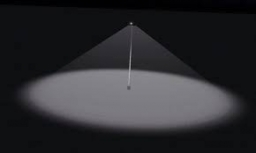We would be pleased if you find an error in the word problem, spelling mistakes, or inaccuracies and send it to us. Thank you!Tips to related online calculators
Do you want to calculate least common multiple two or more numbers?
Do you want to perform natural numbers division - find the quotient and remainder?

#### You need to know the following knowledge to solve this word math problem:

We encourage you to watch this tutorial video on this math problem:

## Next similar math problems:

• Four poplarsFour poplars are growing along the way. The distances between them are 35 m, 14 m, and 91 m. At least how many poplars need to be dropped to create the same spacing between the trees? How many meters will it be?
• Trees in alleyThere are four trees in the alley between which the distances are 35m, 15m and 95m. Trees must be laid in the spaces so that the distance is the same and the maximum. How many trees will they put in and what will be the distance between them?
• Glass panelA rectangular glass panel with dimensions of 72 cm and 96 cm will cut the glazier on the largest square possible. What is the length of the side of each square? How many squares does the glazier cut?
• GardenThe garden has the shape of a rectangle measuring 19m20cm and 21m60cm. Mr. Novák will fence it. It wants the distance between adjacent pillars to be at least two meters and a maximum of three meters. He would also like the distances between the adjacent p
• PlumberPlumber had to cut the metal strip with dimensions 380 cm and 60 cm to the largest squares so that no waste. Calculate the length of the sides of a square. How many squares cut it?
• Paving - jointsWe are paving with rectangular pavement 18 cm × 24 cm was placed side by side in height in a row and in the second row in width etc. How many times will the joints meet at a distance 10 m?
• Apples and pearsMom divided 24 apples and 15 pears to children. Each child received the same number of apples and pears - same number as his siblings. How many apples (j=?) and pears (h=?) received each child?
• School year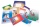At the beginning of the school year, 396 notebooks and 252 textbooks are ready to be distributed in the classroom. All pupils receive the same number of notebooks and the same amount of textbooks. How many pupils are there in the class if you know that th
• Lcm 2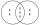Create the smallest possible number that is divisible by numbers 5,8,9,4,3
• Paper squaresThe paper rectangle measuring 69 cm and 46 cm should be cut into as many squares as possible. Calculate the lengths of squares and their number.
• Clock's gears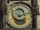In the clock machine, three gears fit together. The largest has 168 teeth, the middle 90 teeth, and the smallest 48 teeth. The middle wheel turns around its axis in 90 seconds. How many times during the day do all the gears meet in the starting position?
• TilesHall has dimensions 325 &time; 170 dm. What is the largest size of square tiles that can be entire hall tiled and how many we need them?
• Three Titanics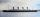Three steamers sailed from the same port on the same day. The first came back on the third day, fourth 4th day and the third returned sixth day. How many days after leaving the steamers met again in the harbor?
• The port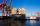Four ships Berthed in the port. Together sail from a port. The first ship will return to port every two weeks, second ship after 4 weeks, third after 8 weeks and fourth ship after 12 weeks. After how many weeks all the boats gather at the port?
• Tailor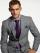From the rest of the cloth tailor could cut off either 3 m in men's suits without vest or 3.6 m with vest. What shortest possible length could the rest of the cloth have? How many suits a) without a vest b) with vest could make the tailor from the rest of
• GroupsIn the 6th class there are 60 girls and 72 boys. We want to divide them into groups so that the number of girls and boys is the same. How many groups can you create? How many girls will be in the group?
• Reminder and quotientThere are given numbers A = 135, B = 315. Find the smallest natural number R greater than 1 so that the proportions R:A, R:B are with the remainder 1.